您可以捐助，支持我们的公益事业。 1元 10元 50元 认证码：必填求知 文章 文库 Lib 视频 iPerson 课程 认证 咨询 工具 讲座 Modeler Code要资料订阅捐助
python计算机视觉：图像边缘检测

 377 次浏览 评价： 好 中 差
2020-8-24

 编辑推荐: 本文主要介绍了图像导数 ，梯度，边缘信息及Python实现卷积及Prewitt算子的边缘检测等内容 。 本文来自于 博客园，由火龙果软件Anna编辑，推荐。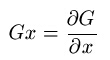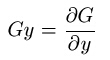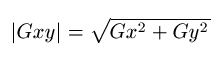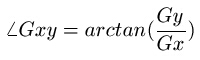x方向的差分：Gx(n,y) = G(n,y)-G(n-1,y)

y方向的差分：Gy(x,n) = G(x,n)-G(x,n-1)

x方向的Prewitt算子为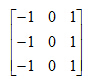y方向的Prewitt算子为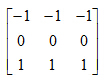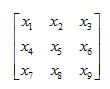x5处的x方向导数为x3+x6+x9-x1-x4-x7Python实现卷积及Prewitt算子的边缘检测

 复制代码 import numpy as np from PIL import Image def imconv(image_array,suanzi): '''计算卷积 参数 image_array 原灰度图像矩阵 suanzi 算子 返回 原图像与算子卷积后的结果矩阵 ''' image = image_array.copy() # 原图像矩阵的深拷贝 dim1,dim2 = image.shape # 对每个元素与算子进行乘积再求和(忽略最外圈边框像素) for i in range(1,dim1-1): for j in range(1,dim2-1): image[i,j] = (image_array[(i-1):(i+2), (j-1):(j+2)]*suanzi).sum() # 由于卷积后灰度值不一定在0-255之间，统一化成0-255 image = image*(255.0/image.max()) # 返回结果矩阵 return image

 import numpy as np import matplotlib.pyplot as plt # x方向的Prewitt算子 suanzi_x = np.array([[-1, 0, 1], [ -1, 0, 1], [ -1, 0, 1]]) # y方向的Prewitt算子 suanzi_y = np.array([[-1,-1,-1], [ 0, 0, 0], [ 1, 1, 1]]) # 打开图像并转化成灰度图像 image = Image.open("pika.jpg").convert("L") # 转化成图像矩阵 image_array = np.array(image) # 得到x方向矩阵 image_x = imconv(image_array,suanzi_x) # 得到y方向矩阵 image_y = imconv(image_array,suanzi_y) # 得到梯度矩阵 image_xy = np.sqrt(image_x**2+image_y**2) # 梯度矩阵统一到0-255 image_xy = (255.0/image_xy.max())*image_xy # 绘出图像 plt.subplot(2,2,1) plt.imshow(image_array,cmap=cm.gray) plt.axis("off") plt.subplot(2,2,2) plt.imshow(image_x,cmap=cm.gray) plt.axis("off") plt.subplot(2,2,3) plt.imshow(image_y,cmap=cm.gray) plt.axis("off") plt.subplot(2,2,4) plt.imshow(image_xy,cmap=cm.gray) plt.axis("off") plt.show()

Prewitt算子 的结果如下图所示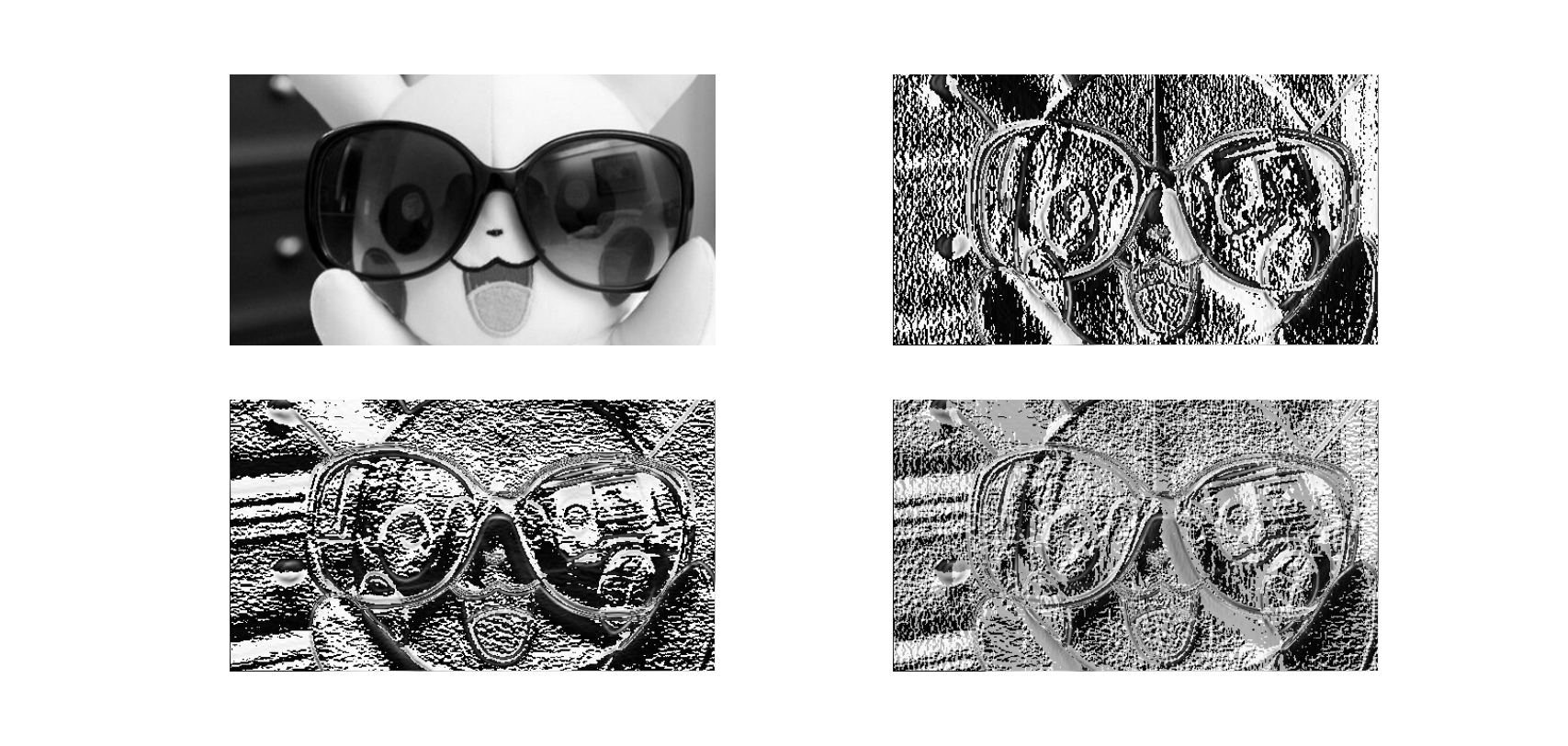Sobel算子与Prewitt比较类似，但是它比Prewitt算子要好一些。

x方向的Sobel算子为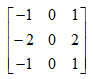y方向的Sobel算子为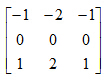python代码只需要将上面代码中的Prewitt算子改成Sobel算子即可。

 # x方向的Sobel算子 suanzi_x = np.array([[-1, 0, 1], [ -2, 0, 2], [ -1, 0, 1]]) # y方向的Sobel算子 suanzi_y = np.array([[-1,-2,-1], [ 0, 0, 0], [ 1, 2, 1]])

Sobel算子 的结果如下图所示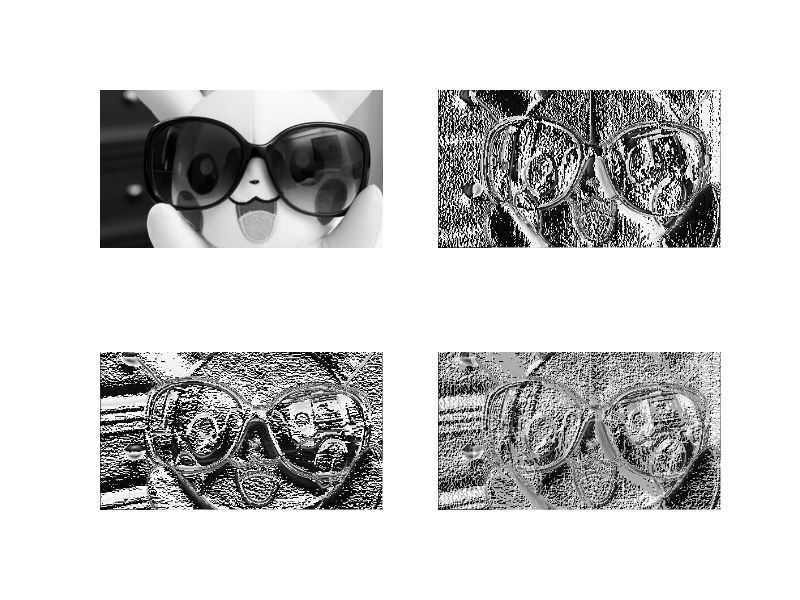Laplace算子是一个二阶导数的算子，它实际上是一个x方向二阶导数和y方向二阶导数的和的近似求导算子。

Laplace算子为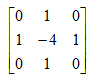Laplace还有一种扩展算子为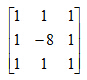convolve()的第一个参数是原图像矩阵，第二个参数为卷积算子，然后指定关键字参数mode="same"(输出矩阵大小和原图像矩阵相同)。

 import numpy as np from PIL import Image import matplotlib.pyplot as plt import matplotlib.cm as cm import scipy.signal as signal # 导入sicpy的signal模块 # Laplace算子 suanzi1 = np.array([[0, 1, 0], [1,-4, 1], [0, 1, 0]]) # Laplace扩展算子 suanzi2 = np.array([[1, 1, 1], [1,-8, 1], [1, 1, 1]]) # 打开图像并转化成灰度图像 image = Image.open("pika.jpg").convert("L") image_array = np.array(image) # 利用signal的convolve计算卷积 image_suanzi1 = signal.convolve2d(image_array,suanzi1,mode="same") image_suanzi2 = signal.convolve2d(image_array,suanzi2,mode="same") # 将卷积结果转化成0~255 image_suanzi1 = (image_suanzi1/float (image_suanzi1.max()))*255 image_suanzi2 = (image_suanzi2/float (image_suanzi2.max()))*255 # 为了使看清边缘检测结果，将大于灰度平均值的灰度变成255(白色) image_suanzi1[image_suanzi1> image_suanzi1.mean()] = 255 image_suanzi2[image_suanzi2> image_suanzi2.mean()] = 255 # 显示图像 plt.subplot(2,1,1) plt.imshow(image_array,cmap=cm.gray) plt.axis("off") plt.subplot(2,2,3) plt.imshow(image_suanzi1,cmap=cm.gray) plt.axis("off") plt.subplot(2,2,4) plt.imshow(image_suanzi2,cmap=cm.gray) plt.axis("off") plt.show()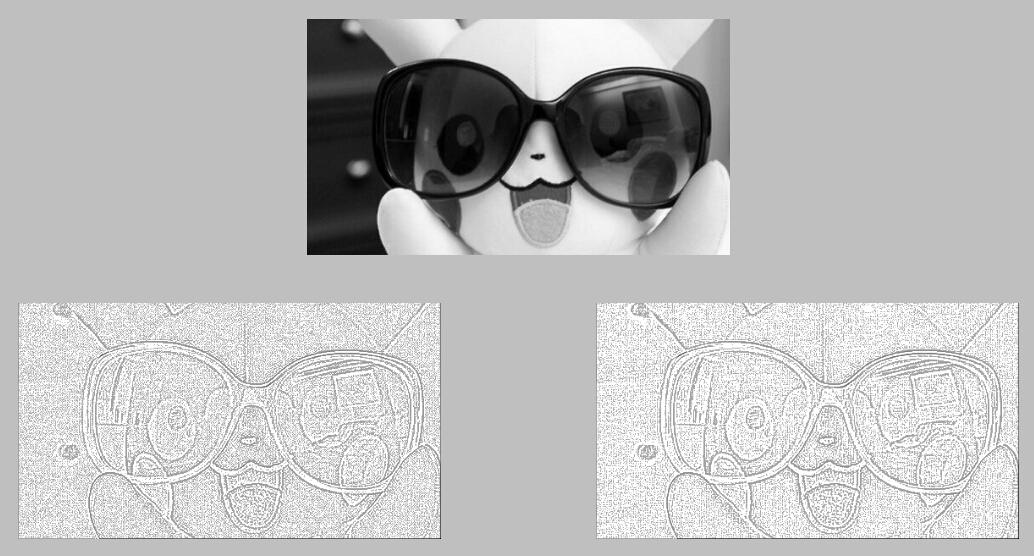python程序如下

 import numpy as np from PIL import Image import matplotlib.pyplot as plt import matplotlib.cm as cm import scipy.signal as signal # 生成高斯算子的函数 def func(x,y,sigma=1): return 100*(1/(2*np.pi*sigma)) *np.exp(-((x-2)**2+(y-2)**2)/(2.0*sigma**2)) # 生成标准差为5的5*5高斯算子 suanzi1 = np.fromfunction(func,(5,5),sigma=5) # Laplace扩展算子 suanzi2 = np.array([[1, 1, 1], [1,-8, 1], [1, 1, 1]]) # 打开图像并转化成灰度图像 image = Image.open("pika.jpg").convert("L") image_array = np.array(image) # 利用生成的高斯算子与原图像进行卷积对图像进行平滑处理 image_blur = signal.convolve2d(image_array, suanzi1, mode= "same") # 对平滑后的图像进行边缘检测 image2 = signal.convolve2d(image_blur, suanzi2, mode="same") # 结果转化到0-255 image2 = (image2/float(image2.max()))*255 # 将大于灰度平均值的灰度值变成255（白色），便于观察边缘 image2[image2>image2.mean()] = 255 # 显示图像 plt.subplot(2,1,1) plt.imshow(image_array,cmap=cm.gray) plt.axis("off") plt.subplot(2,1,2) plt.imshow(image2,cmap=cm.gray) plt.axis("off") plt.show()377 次浏览     评价： 好 中 差订阅捐助
 相关文章 手机软件测试用例设计实践 手机客户端UI测试分析 iPhone消息推送机制实现与探讨 Android手机开发（一）
 相关文档 Android_UI官方设计教程 手机开发平台介绍 android拍照及上传功能 Android讲义智能手机开发
 相关课程 Android高级移动应用程序 Android系统开发 Android应用开发 手机软件测试
 最新课程计划

 最新文章 简述Matplotlib Python三维绘图--Matplotlib Python数据清洗实践 PyTorch实战指南 Python爬虫与数据可视化 最新课程 Python应用开发最佳实践 Python+数据分析+tensorflow Python 编程方法和应用开发 人工智能+Python＋大数据 Python及数据分析 成功案例 某通信设备企业 Python数据分析与挖掘 某银行 人工智能+Python+大数据 某领先数字地图提供商 Python数据分析与机器学习 北京 Python及数据分析 某金融公司 Python编程方法与实践培训